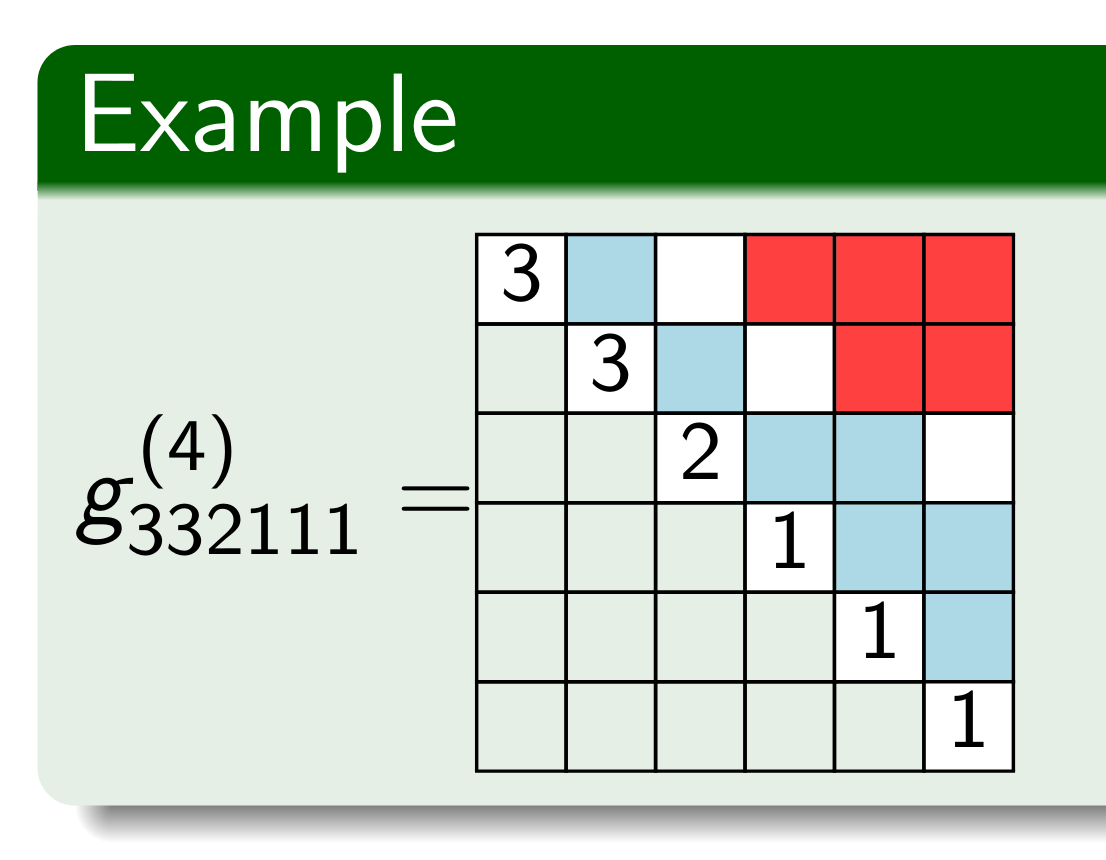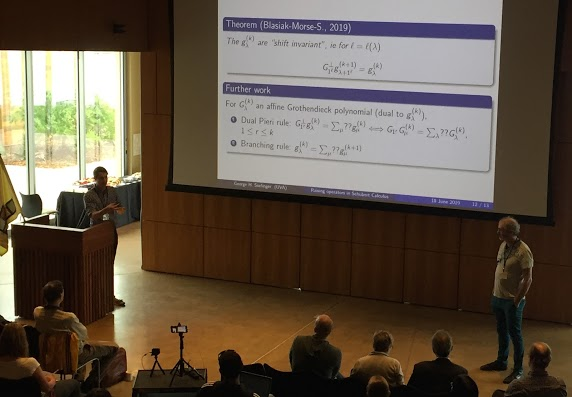# Garsiafest Lightning Talk: Raising Operators in Schubert CalculusThis past week I had the opportunity to give my first public research presentation as a graduate student at Adriano Garsia’s 90th birthday conference, Garsiafest, in San Diego at the Scripps Seaside Forum. My presentation mainly expanded on the information from my MAAGC Poster, but now one of the main conjectures is a theorem! You can look at the slides here. Eventually, they will also post a video of my talk on the conference website. It was truly an honor to present my work to so many people in my area and I look forward to presenting more work in the future.## What is a Lightning Talk?

A lightning talk is a very short talk (this one was 8 minutes long) and, as a result, requires that the speaker only conveys the most critical information. As far as I can tell, the concept of a lightning talk originated in the programming community, but I think the idea translates well into math conferences when most of the audience is in the same field as the presenter.

## Some Exposition on the Talk

There is a good blog post on Schubert calculus background on Maria Gillespie’s blog, [Mathematical Gemstones] for a more in-depth summary of the classical background motivating a lot of this work. However, in my work, I am trying to describe representatives for various Schubert classes with families symmetric functions, especially using raising operators.

Some known examples of raising operators in Schubert classes are

1. Schur functions given by the Jacobi-Trudi identity, $$s_\lambda(x) = \prod_{i < j} (1-R_{ij}) h_\lambda$$;
2. $$k$$-Schur functions given by a Catalan formula, $$s_\lambda^{(k)}(x;1) = \prod_{(i,j \in \Delta^+ \setminus \Delta^{(k)}(\lambda))} (1-R_{ij}) h_\lambda$$, which give the homology Schubert representatives for the “affine Grassmannian”;
3. dual Grothendieck polynomials, $$g_\lambda(x) = \prod_{i < j} (1-R_{ij}) Kh_\lambda$$ where $$Kh_m^{(r)} = \sum_{i=0}^m \binom{r+i-1}{i} h_{m-i}$$ and $$Kh_\lambda = Kh_{\lambda_1}^{(0)} Kh_{\lambda_2}^{(1)} \cdots Kh_{\lambda_\ell}^{(\ell-1)}$$.

Recently, we have also proven a formula for the “dual affine Grothendieck polynomials”, or sometimes called $$K$$-$$k$$-Schur functions, $$g_\lambda^{(k)}(x)$$. These polynomials represent the “$$K$$-homology Schubert classes” of the affine Grassmannian. We have shown they are of the form $$g_\lambda^{(k)} = \prod_{(i,j) \in \Delta^{(k+1)}(\lambda)}(1-L_j)\prod_{(i,j) \in \Delta^+ \setminus \Delta^{(k)}(\lambda)}(1-R_{ij}) Kh_\lambda$$, which looks a lot like a hybrid of the dual Grothendieck polynomials and the $$k$$-Schur functions with the extra ingredient of the lowering operators.

Furthermore, we proved the $$g_\lambda^{(k)}$$ functions have a property called “shift invariance” similar to the $$k$$-Schur functions. Essentially, this property tells you that the dual Pieri rule for $$g_\lambda^{(k)}$$ determines the coefficients $$c_{\lambda,\mu}^{(k)}$$, called “branching coefficients,” in the expansion $$g_\lambda^{(k)} = \sum_\mu c_{\lambda \mu}^{(k)} g_{\mu}^{(k+1)}$$. However, as of now, no combinatorial description of the dual Pieri rule coefficients nor of the branching rule coefficients is known. However, after my talk, Mark Shimozono informed me that the dual Pieri rule coefficients are known to satisfy (a notion of) positivity from a paper by Baldwin and Kumar. Then, using the property of shift invariance, we immediately answer Conjecture 7.20(c) of Lam, Schilling, and Shimozono in their paper $$K$$-Theory Schubert Calculus of the Affine Grassmannian. So, I have discovered that presenting at conferences can be really helpful sometimes because an audience member may tell you to look at something useful you missed!

However, we are not satisfied with merely answering positivity questions for these coefficients, but would like to be able to describe them combinatorially; so far, we have made some good progress using the raising operator techniques and continue to work on this problem.

1. S. Baldwin, S. Kumar, Positivity in $$T$$-equivariant $$K$$-theory of flag varieties associated to Kac-Moody groups II, Represent. Theory (2017)), 35–60.
2. J. Blasiak, J. Morse, A. Pun, D. Summers, Catalan Functions and $$k$$-Schur Positivity, Journal of the AMS (2019).
3. T. Lam, A. Schilling, M. Shimozono, $$K$$-theory Schubert calculus of the affine Grassmannian, Compositio Math. (2010), 811–852.
4. J. Morse, Combinatorics of the $$K$$-theory of affine Grassmannians, Advances in Mathematics (2011).

Date published: Monday, June 24, 2019